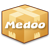version: 2.1.3

### count

Count the number of rows from the table.

count(\$table, \$where)
• ##### table [string]

The table name.

• ##### where (optional) [array]

The WHERE clause to filter records.

count(\$table, \$join, \$column, \$where)
• ##### table [string]

The table name.

• ##### join [array]

Table relativity for table joining.

• ##### column [string]

The target column will be counted.

• ##### where (optional) [array]

The WHERE clause to filter records.

Return: [number] The number of rows.
The type of returned data is number.
```\$count = \$database->count("account", [
"gender" => "female"
]);

echo "We have " . \$count . " female users.";```